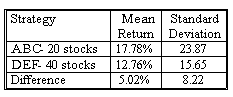### CFA Practice Question

There are 985 practice questions for this topic.

### CFA Practice Question

The table below is a summary of results obtained by an analyst who examined the investment strategy in 20 stocks with the highest yields in the ABC Average versus a buy-and-hold strategy in all 40 stocks of the DEF. The study period is 40 years.We are testing the null and alternative hypotheses consistent with a two-sided test that the mean difference between the ABC and DEF strategies equals 0. The decision made in this hypothesis is ______.

A. to reject the null hypothesis
B. to reject the alternative hypothesis
C. that a Type I error has occurred

We look in the t-table at 39 degrees of freedom and the 0.005 column and we find 1.685. We reject the null hypothesis if t > 1.685 or t < -1.685.

t39 = 5.02/(8.22/401/2) = 3.862

3.862 is greater than 1.685 and thus we reject the null hypothesis. Note: We use the differences because our test concerns itself with the mean difference.

User Comment
kaliokale Why need to use root(40) but not root(20)?
zhizhima because n=40 years
Rotigga We assume population standard deviations are unknown and unequal
Ho: mu1-mu2=0
Ha: mu1-mu2<>0
Let Sh1=s1^2/n1; Same with Sh2; n1=20; n2=40; s1=23.87; s2=15.65
Df=[Sh1+Sh2]^2/[Sh1^2/n1 + Sh2^2/n2]
Sh1=(23.87)^2/20=28.48845
Sh2=(15.65)^2/40=6.1230625
Df=1197.984/[40.5807145+0.9372974]=28.8545 ~ 29
Sp^2=[(n1-1)*s1^2 + (n2-1)*s2^2]/(n1+n2-2) is the pooled estimator
Sp^2=[19*23.87^2 + 39*15.65^2]/(58)=351.3403207
T=(xbar1-xbar2)-(mu1-mu2)/[sp^2/n1 + sp^2/n2]^0.5
T value=(17.78-12.76)-(0)/5.13327615=0.97793297
Critical value (two-sided alpha/2=0.025, Df=29)=2.04523
Do not reject Ho

Anybody?
chamad I tought 80 was the maximum in the table. Are you sure about 45df.

I' completely lost. why t39. Don't we have 58 (20 + 40 -2) df?...Help!
Xocrevilo This is another question which I can readily admit to not understanding at all, but the method in the answer is clear and simple, which should be enough information to get a close enough answer if a question like this one does come up in the exam. Rottiga's methodology is impressive, but I doubt that most of us will be able to commit so much time to working on just one question!
sanyukta where did o.o5 come from?
jd2442424 The solution is valid only if the mean and standard deviation are of the differences between the two samples. This is not stated in the question and is a dangerous assumption to make given that Rotigga is correct in the solution that laid out in both the curriculum and the notes.
dcfa since ABC and DEF are not independent, a paired comparison test is used here -
test statistic t = d-bar - mu-diff / sd/sqrt(n)
sd= sum(di-d-bar/n-1)^1/2 but sd is given 8.22
d-bar=5.02
thus t=5.02-0 /8.22/sqrt(40)
johntan1979 I also attacked this question the same method Rotigga used, and then realized from reading the answer that the SD for the difference is ALREADY GIVEN! No need to calculate t, sp^2 or df. Besides, n is 40 years, not the number of stocks.

And by the way, if the significance level is not given, we always assume it's 5%.
sgossett86 in the formula for mean differences it says to take square root of standard deviation divided by N

in the solution we take the standard deviation divided by square root of n
sgossett86 DCFA is wrong about using paired comparison test because the samples are "definitely not independent". In the next section, question 2 or 3 states that paired comparison tests are only used when comparing the means of INDEPENDENT samples...
Shaan23 I also used the paired difference and not the mean difference test. Portfolio theory states stocks are dependent and not independent <--- that Q has arose before. So we cannot use the mean test.

This question works with the paired comparison test pretty easy which is the next section
Marinov If the significance level is 5%, then for a two-tailed test we must surely look at the column 0.025?
dbedford Did we assume that variances were equal because the sample size (40) was the same for both?

I read somewhere online that if sample size was the same to assume standard error was the same for both and I suppose variance is the same also?
Freddie33 The answers assumes that population variance is the same, but this is stated nowhere in the question.